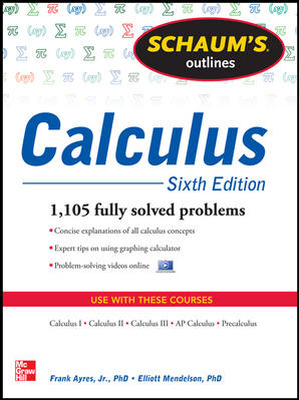# Schaum's Outline of Calculus, 6th Edition

6th Edition
By Frank Ayres and Elliott Mendelson
ISBN10: 0071795537
ISBN13: 9780071795531

## Purchase Options

Students, we’re committed to providing you with high-value course solutions backed by great service and a team that cares about your success. See tabs below to explore options and pricing. Don't forget, we accept financial aid and scholarship funds in the form of credit or debit cards.

### Product

The estimated amount of time this product will be on the market is based on a number of factors, including faculty input to instructional design and the prior revision cycle and updates to academic research-which typically results in a revision cycle ranging from every two to four years for this product. Pricing subject to change at any time.

### Program Details

Linear Coordinate Systems. Absolute Value. Inequalities • Rectangular Coordinate Systems • Lines • Circles • Equations and their Graphs • Functions • Limits • Continuity • The Derivative • Rules for Differentiating Functions • Implicit Differentiation • Tangent and Normal Lines • Law of the Mean. Increasing and Decreasing Functions • Maximum and Minimum Values • Curve Sketching. Concavity. Symmetry • Review of Trigonometry • Differentiation of Trigonometric Functions • Inverse Trigonometric Functions • Rectilinear and Circular Motion • Related Rates • Differentials. Newton’s Method • Antiderivatives • The Definite Integral. Area under a Curve • The Fundamental Theorem of Calculus • The Natural Logarithm • Exponential and Logarithmic Functions • L’Hopital’s Rule • Exponential Growth and Decay • Applications of Integration I: Area and Arc Length • Applications of Integration II: Volume • Techniques of Integration I: Integration by Parts • Techniques of Integration II: Trigonometric Integrands and Trigonometric Substitutions • Techniques of Integration III: Integration by Partial Fractions • Miscellaneous Substitutions • Improper Integrals • Applications of Integration II: Area of a Surface of Revolution • Parametric Representation of Curves • Curvature • Plane Vectors • Curvilinear Motion • Polar Coordinates • Infinite Sequences • Infinite Series • Series with Positive Terms. The Integral Test. Comparison Tests • Alternating Series. Absolute and Conditional Convergence. The Ratio Test • Power Series • Taylor and Maclaurin Series. Taylor’s Formula with Remainder • Partial Derivatives • Total Differential. Differentiability. Chain Rules • Space Vectors • Surface and Curves in Space • Directional Derivatives. Maximum and Minimum Values • Vector Differentiation and Integration • Double and Iterated Integrals • Centroids and Moments of Inertia of Plane Areas • Double Integration Applied to Volume under a Surface and the Area of a Curved Surface • Triple Integrals • Masses of Variable Density • Differential Equations of First and Second Order